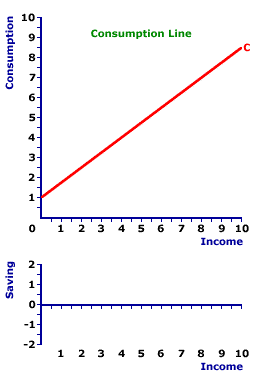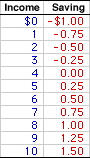Monday  December 9, 2019
 AmosWEB means Economics with a Touch of Whimsy!FINANCIAL WEALTH, AGGREGATE EXPENDITURES DETERMINANT: One of several specific aggregate expenditures determinants assumed constant when the aggregate expenditures line is constructed, and that shifts the aggregate expenditures line when it changes. An increase in financial wealth causes an increase (upward shift) of the aggregate expenditures line. A decrease in financial wealth causes a decrease (downward shift) of the aggregate expenditures line. Other notable aggregate expenditures determinants include consumer confidence, federal deficit, inflationary expectations, and exchange rates.DERIVATION, SAVING LINE:

A saving line, a graphical depiction of the relation between household sector saving and income, can be derived from the consumption line. The saving line can also be derived by plotting the saving-income information from a saving schedule or using the slope and intercept values of the saving function. However, derivation from the consumption line emphasis the connection between consumption and income--that the household sector uses a portion of income for consumption and a portion for saving.
The saving line can be derived in a variety of ways. The most common method is directly from the consumption line. It can also be derived by plotting the saving-income combinations from a saving schedule. Lastly, the saving line can be derived by plotting the mathematical specification of a saving function using slope and intercept.
From Consumption
To SavingThe saving line, also termed propensity-to-save line or saving function, is most often derived from the consumption line. This derivation is based on the observation that income is divided between consumption and saving. Any income not used for consumption is diverted to saving.

The exhibit to the right can be used to derived the saving line from the consumption line. The top panel presents a standard consumption line. This line is a plot of a consumption function specified as:

 C = 1 + 0.75Y
where: C is consumption expenditures and Y is income (national or disposable). The intercept (or autonomous consumption) is \$1 trillion and the slope (or induced consumption, which is also the marginal propensity to consume) is 0.75.

The key to deriving the saving line is the addition of a 45-degree line. This line indicates every point in the upper panel in which consumption measured on the vertical axis is exactly equal to income measured on the horizontal axis. Such a line has a slope of one and thus exactly bisects the 90-degree angle formed by the vertical and horizontal axes. Click the [45-degree Line] button to make this addition.

Because the 45-degree line indicates equality between consumption and income, when compared to the consumption line it can also be used to indicate saving. In particular, the vertical difference between the 45-degree line and the consumption line is saving. If the 45-degree line lies below the consumption line, as is the case for relatively low levels of income, then saving is negative. If the 45-degree line lies above the consumption line, as is the case for relatively high levels of income, then saving is positive.

The saving line can be derived by transferring the vertical distance between the 45-degree line and the consumption line from the top panel to the bottom panel of the exhibit. The bottom panel measures saving on the vertical axis and income on the horizontal axis. A click of the [Saving Line] works through the derivation. Note how the vertical distance in the top panel is first identified, then this value is plotted in the bottom panel.

Once all points are plotted, the result is the red saving line labeled S. The two features of the saving line are slope and intercept.

• Slope: The slope is positive, but less than one. In fact, the slope of the saving line is numerically equal to the marginal propensity to save (MPS). In this case the slope is equal to 0.25. The positive slope reflects induced saving--more income means more saving. Click the [Slope] button to illustrate.

• Intercept: The saving line intersects the vertical axis at a value of -\$1 trillion. This intersection point is autonomous saving--saving unrelated to income. Click the [Intercept] button to illustrate.
Saving ScheduleWhile the derivation of the saving line from the consumption line is the most common approach, the saving line can also be derived in a couple of other ways.
• One: Supply Schedule: One alternative is simply to plot the saving-income values that make up a saving schedule, such as the one presented to the right. Once the points are plotted, they can be connected to form the saving line. A quick comparison of the numbers in the schedule and the saving line derived above should indicate the consistency of either approach.

• Two: Supply Function: Another alternative is to plot the saving line using the saving function. The two key bits of information needed for this task are the intercept and the slope. The following linear saving function can be used to illustrate.
 S = - 1 + 0.25Y
where: S is saving and Y is income (national or disposable). The intercept (or autonomous saving) is \$1 trillion and the slope (or induced saving, which is also the marginal propensity to save) is 0.25.

The saving line can be constructed by simply drawing a line with slope 0.25 that emerges for the vertical axis at intercept \$1. A quick calculation of the slope and intercept of the saving line derived above should indicate the consistency of this approach, too.

 <= DERIVATION, PRODUCTION POSSIBILITIES CURVE DERIVED DEMAND =>Recommended Citation:

DERIVATION, SAVING LINE, AmosWEB Encyclonomic WEB*pedia, http://www.AmosWEB.com, AmosWEB LLC, 2000-2019. [Accessed: December 9, 2019].

Check Out These Related Terms...

Or For A Little Background...

And For Further Study...
Search Again?BEIGE MUNDORTLE[What's This?] Today, you are likely to spend a great deal of time at a crowded estate auction hoping to buy either storage boxes for your winter clothes or several magazines on time travel. Be on the lookout for a thesaurus filled with typos.Your Complete ScopeFrancis Bacon (1561-1626), a champion of the scientific method, died when he caught a severe cold while attempting to preserve a chicken by filling it with snow."If you don't make mistakes, you aren't really trying."-- Coleman Hawkings,musicianNPVNet Present ValueA PEDestrian's Guide Xtra CreditTell us what you think about AmosWEB. Like what you see? Have suggestions for improvements? Let us know. Click the User Feedback link.| | | | | | | | | | |
| | | |

Thanks for visiting AmosWEB